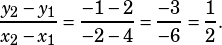If the xy-plane, a line contains the points (4,2), (-2,-1) and (k,5). What is the value of k?

A 6
B 7
C 8
D 9
E 10

Use the first two points to find the slope of the line.From there, you can either use the slope formula again to solve for k, or you can just count up and over from (4, 2). A line that goes through (4, 2) and has a slope of 1/2 will go like this:

(4, 2)
(6, 3)
(8, 4)
(10, 5)